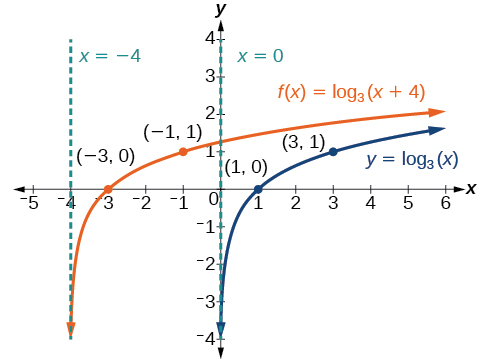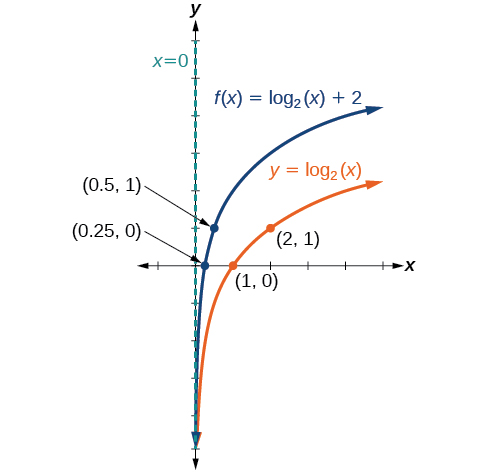# 6.4 Graphs of logarithmic functions  (Page 4/8)

 Page 4 / 8

Sketch a graph of $\text{\hspace{0.17em}}f\left(x\right)={\mathrm{log}}_{3}\left(x+4\right)\text{\hspace{0.17em}}$ alongside its parent function. Include the key points and asymptotes on the graph. State the domain, range, and asymptote.The domain is $\text{\hspace{0.17em}}\left(-4,\infty \right),$ the range $\text{\hspace{0.17em}}\left(-\infty ,\infty \right),$ and the asymptote $\text{\hspace{0.17em}}x=–4.$

## Graphing a vertical shift of y = log b ( x )

When a constant $\text{\hspace{0.17em}}d\text{\hspace{0.17em}}$ is added to the parent function $\text{\hspace{0.17em}}f\left(x\right)={\mathrm{log}}_{b}\left(x\right),$ the result is a vertical shift     $\text{\hspace{0.17em}}d\text{\hspace{0.17em}}$ units in the direction of the sign on $\text{\hspace{0.17em}}d.\text{\hspace{0.17em}}$ To visualize vertical shifts, we can observe the general graph of the parent function $\text{\hspace{0.17em}}f\left(x\right)={\mathrm{log}}_{b}\left(x\right)\text{\hspace{0.17em}}$ alongside the shift up, $\text{\hspace{0.17em}}g\left(x\right)={\mathrm{log}}_{b}\left(x\right)+d\text{\hspace{0.17em}}$ and the shift down, $\text{\hspace{0.17em}}h\left(x\right)={\mathrm{log}}_{b}\left(x\right)-d.$ See [link] .

## Vertical shifts of the parent function y = log b ( x )

For any constant $\text{\hspace{0.17em}}d,$ the function $\text{\hspace{0.17em}}f\left(x\right)={\mathrm{log}}_{b}\left(x\right)+d$

• shifts the parent function $\text{\hspace{0.17em}}y={\mathrm{log}}_{b}\left(x\right)\text{\hspace{0.17em}}$ up $\text{\hspace{0.17em}}d\text{\hspace{0.17em}}$ units if $\text{\hspace{0.17em}}d>0.$
• shifts the parent function $\text{\hspace{0.17em}}y={\mathrm{log}}_{b}\left(x\right)\text{\hspace{0.17em}}$ down $\text{\hspace{0.17em}}d\text{\hspace{0.17em}}$ units if $\text{\hspace{0.17em}}d<0.$
• has the vertical asymptote $\text{\hspace{0.17em}}x=0.$
• has domain $\text{\hspace{0.17em}}\left(0,\infty \right).$
• has range $\text{\hspace{0.17em}}\left(-\infty ,\infty \right).$

Given a logarithmic function with the form $\text{\hspace{0.17em}}f\left(x\right)={\mathrm{log}}_{b}\left(x\right)+d,$ graph the translation.

1. Identify the vertical shift:
• If $\text{\hspace{0.17em}}d>0,$ shift the graph of $\text{\hspace{0.17em}}f\left(x\right)={\mathrm{log}}_{b}\left(x\right)\text{\hspace{0.17em}}$ up $\text{\hspace{0.17em}}d\text{\hspace{0.17em}}$ units.
• If $\text{\hspace{0.17em}}d<0,$ shift the graph of $\text{\hspace{0.17em}}f\left(x\right)={\mathrm{log}}_{b}\left(x\right)$ down $\text{\hspace{0.17em}}d\text{\hspace{0.17em}}$ units.
2. Draw the vertical asymptote $\text{\hspace{0.17em}}x=0.$
3. Identify three key points from the parent function. Find new coordinates for the shifted functions by adding $\text{\hspace{0.17em}}d\text{\hspace{0.17em}}$ to the $\text{\hspace{0.17em}}y\text{\hspace{0.17em}}$ coordinate.
4. Label the three points.
5. The domain is $\text{\hspace{0.17em}}\left(0,\infty \right),$ the range is $\text{\hspace{0.17em}}\left(-\infty ,\infty \right),$ and the vertical asymptote is $\text{\hspace{0.17em}}x=0.$

## Graphing a vertical shift of the parent function y = log b ( x )

Sketch a graph of $\text{\hspace{0.17em}}f\left(x\right)={\mathrm{log}}_{3}\left(x\right)-2\text{\hspace{0.17em}}$ alongside its parent function. Include the key points and asymptote on the graph. State the domain, range, and asymptote.

Since the function is $\text{\hspace{0.17em}}f\left(x\right)={\mathrm{log}}_{3}\left(x\right)-2,$ we will notice $\text{\hspace{0.17em}}d=–2.\text{\hspace{0.17em}}$ Thus $\text{\hspace{0.17em}}d<0.$

This means we will shift the function $\text{\hspace{0.17em}}f\left(x\right)={\mathrm{log}}_{3}\left(x\right)\text{\hspace{0.17em}}$ down 2 units.

The vertical asymptote is $\text{\hspace{0.17em}}x=0.$

Consider the three key points from the parent function, $\text{\hspace{0.17em}}\left(\frac{1}{3},-1\right),$ $\left(1,0\right),$ and $\text{\hspace{0.17em}}\left(3,1\right).$

The new coordinates are found by subtracting 2 from the y coordinates.

Label the points $\text{\hspace{0.17em}}\left(\frac{1}{3},-3\right),$ $\left(1,-2\right),$ and $\text{\hspace{0.17em}}\left(3,-1\right).$

The domain is $\text{\hspace{0.17em}}\left(0,\infty \right),$ the range is $\text{\hspace{0.17em}}\left(-\infty ,\infty \right),$ and the vertical asymptote is $\text{\hspace{0.17em}}x=0.$

The domain is $\text{\hspace{0.17em}}\left(0,\infty \right),$ the range is $\text{\hspace{0.17em}}\left(-\infty ,\infty \right),$ and the vertical asymptote is $\text{\hspace{0.17em}}x=0.$

Sketch a graph of $\text{\hspace{0.17em}}f\left(x\right)={\mathrm{log}}_{2}\left(x\right)+2\text{\hspace{0.17em}}$ alongside its parent function. Include the key points and asymptote on the graph. State the domain, range, and asymptote.The domain is $\text{\hspace{0.17em}}\left(0,\infty \right),$ the range is $\text{\hspace{0.17em}}\left(-\infty ,\infty \right),$ and the vertical asymptote is $\text{\hspace{0.17em}}x=0.$

## Graphing stretches and compressions of y = log b ( x )

When the parent function $\text{\hspace{0.17em}}f\left(x\right)={\mathrm{log}}_{b}\left(x\right)\text{\hspace{0.17em}}$ is multiplied by a constant $\text{\hspace{0.17em}}a>0,$ the result is a vertical stretch    or compression of the original graph. To visualize stretches and compressions, we set $\text{\hspace{0.17em}}a>1\text{\hspace{0.17em}}$ and observe the general graph of the parent function $\text{\hspace{0.17em}}f\left(x\right)={\mathrm{log}}_{b}\left(x\right)\text{\hspace{0.17em}}$ alongside the vertical stretch, $\text{\hspace{0.17em}}g\left(x\right)=a{\mathrm{log}}_{b}\left(x\right)\text{\hspace{0.17em}}$ and the vertical compression, $\text{\hspace{0.17em}}h\left(x\right)=\frac{1}{a}{\mathrm{log}}_{b}\left(x\right).$ See [link] .

## Vertical stretches and compressions of the parent function y = log b ( x )

For any constant $\text{\hspace{0.17em}}a>1,$ the function $\text{\hspace{0.17em}}f\left(x\right)=a{\mathrm{log}}_{b}\left(x\right)$

• stretches the parent function $\text{\hspace{0.17em}}y={\mathrm{log}}_{b}\left(x\right)\text{\hspace{0.17em}}$ vertically by a factor of $\text{\hspace{0.17em}}a\text{\hspace{0.17em}}$ if $\text{\hspace{0.17em}}a>1.$
• compresses the parent function $\text{\hspace{0.17em}}y={\mathrm{log}}_{b}\left(x\right)\text{\hspace{0.17em}}$ vertically by a factor of $\text{\hspace{0.17em}}a\text{\hspace{0.17em}}$ if $\text{\hspace{0.17em}}0
• has the vertical asymptote $\text{\hspace{0.17em}}x=0.$
• has the x -intercept $\text{\hspace{0.17em}}\left(1,0\right).$
• has domain $\text{\hspace{0.17em}}\left(0,\infty \right).$
• has range $\text{\hspace{0.17em}}\left(-\infty ,\infty \right).$

#### Questions & Answers

A laser rangefinder is locked on a comet approaching Earth. The distance g(x), in kilometers, of the comet after x days, for x in the interval 0 to 30 days, is given by g(x)=250,000csc(π30x). Graph g(x) on the interval [0, 35]. Evaluate g(5)  and interpret the information. What is the minimum distance between the comet and Earth? When does this occur? To which constant in the equation does this correspond? Find and discuss the meaning of any vertical asymptotes.
Kaitlyn Reply
The sequence is {1,-1,1-1.....} has
amit Reply
circular region of radious
Kainat Reply
how can we solve this problem
Joel Reply
Sin(A+B) = sinBcosA+cosBsinA
Eseka Reply
Prove it
Eseka
Please prove it
Eseka
hi
Joel
June needs 45 gallons of punch. 2 different coolers. Bigger cooler is 5 times as large as smaller cooler. How many gallons in each cooler?
Arleathia Reply
7.5 and 37.5
Nando
find the sum of 28th term of the AP 3+10+17+---------
Prince Reply
I think you should say "28 terms" instead of "28th term"
Vedant
the 28th term is 175
Nando
192
Kenneth
if sequence sn is a such that sn>0 for all n and lim sn=0than prove that lim (s1 s2............ sn) ke hole power n =n
SANDESH Reply
write down the polynomial function with root 1/3,2,-3 with solution
Gift Reply
if A and B are subspaces of V prove that (A+B)/B=A/(A-B)
Pream Reply
write down the value of each of the following in surd form a)cos(-65°) b)sin(-180°)c)tan(225°)d)tan(135°)
Oroke Reply
Prove that (sinA/1-cosA - 1-cosA/sinA) (cosA/1-sinA - 1-sinA/cosA) = 4
kiruba Reply
what is the answer to dividing negative index
Morosi Reply
In a triangle ABC prove that. (b+c)cosA+(c+a)cosB+(a+b)cisC=a+b+c.
Shivam Reply
give me the waec 2019 questions
Aaron Reply

### Read also:

#### Get the best Algebra and trigonometry course in your pocket!

Source:  OpenStax, Algebra and trigonometry. OpenStax CNX. Nov 14, 2016 Download for free at https://legacy.cnx.org/content/col11758/1.6
Google Play and the Google Play logo are trademarks of Google Inc.

Notification Switch

Would you like to follow the 'Algebra and trigonometry' conversation and receive update notifications?ByByByByBy